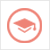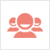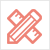﻿ 更多范文-Assignment代写：Arrays – Mean, Median & Mode-51Due留学教育

#### 服务承诺资金托管原创保证实力保障24小时客服使命必达

#### 关于我们

51Due提供Essay，Paper，Report，Assignment等学科作业的代写与辅导，同时涵盖Personal Statement，转学申请等留学文书代写。51Due将让你达成学业目标51Due将让你达成学业目标51Due将让你达成学业目标51Due将让你达成学业目标

#### 名企实习积累工作经验多元化文化交流专业实操技能建立人际资源圈

# Assignment代写：Arrays – Mean, Median & Mode

2018-03-31 来源: 51due教员组 类别: 更多范文

Write a program that will read an unknown number of marks (loops), continuing to ask for marks until a sentinel value of -1 is read. Assume the marks are whole (integer) numbers between 0 and 10. Output an error message for marks outside of this range.

For each valid mark entered, you will keep a count of how many times that mark occurred. For example, if the user enters 5, 7, and 5, then there have been 2 occurrences of the mark 5, and 1 occurrence of the mark 7. This type of data is commonly called frequency data, as we are tracking the frequency of each mark.

You will need an array to store this data:

i. What kind of data will the array store?

ii. How many elements are required for the array?

iii. What is a good, meaningful name for the array?

Once mark entry is completed (using the sentinel value of -1), your program should:

(a) Display a summary of the frequency data. List each possible mark (from 0 to 10) and beside it, display the number of occurrences of that mark.

Sample Input Sample Output

0 Mark Frequency

0 0 2

1 1 2

1 2 1

2 3 2

3 4 0

3 5 0

6 0

7 0

8 0

9 0

10 0

(b) Calculate and display the arithmetic mean of the marks. The mean is the sum of the marks divided by the total number of marks.

Given: 3, 4, 5, 6, 7

Sum is 25

Mean is 25 / 5 = 5

(c) Determine and display the mode of the marks. The mode of a group of values is the value that occurs most often in the group. A group may have more than one mode if more than one value occurs with the maximum frequency. Such a group is said to be multi-modal.

Given: 3, 4, 5, 6, 7

All numbers occur once.

The mode is 3, 4, 5, 6, and 7

Given: 3, 3, 4, 5, 5, 5, 6, 7, 7

The maximum frequency, or number of occurrences, is 3 times for the number 5.

The mode is 5

(d) Determine and display the median of the marks. The median is the middle value from an ordered list of all the values. If there are an odd number of values, the median will be the middle value. If there are even numbers of values, the median is the average of the two values adjacent to the middle.

Given: 3, 4, 5, 6, 7

There are 5 terms (odd), so the median is the middle term.

The median is 5

Given: 3, 3, 3, 4, 4, 4

There are 6 terms (even), so the median is the mean of the two middle terms.

The middle terms are 3 and 4.

The median is (3 + 4) / 2 = 3.5

Given: 3, 3, 3, 3, 4, 4

There are 6 terms (even), so the median is the mean of the two middle terms.

The middle terms are 3 and 3.

The median is (3 + 3) / 2 = 3

Save you file as Median_yourName.java

Allow to REPEAT, 2 decimal digits output

51due留学教育原创版权郑重声明：原创assignment代写范文源自编辑创作，未经官方许可，网站谢绝转载。对于侵权行为，未经同意的情况下，51Due有权追究法律责任。主要业务有assignment代写、essay代写、paper代写、cs代写服务。

51due为留学生提供最好的assignment代写服务，亲们可以进入主页了解和获取更多assignment代写范文 提供作业代写服务，详情可以咨询我们的客服QQ：800020041。